# Improper Fraction - Definition with Examples

The Complete K-5 Math Learning Program Built for Your Child

• 40 Million Kids

Loved by kids and parent worldwide

• 50,000 Schools

Trusted by teachers across schools

• Comprehensive Curriculum

Aligned to Common Core

## Improper Fraction

A fraction denotes a part of a whole or a collection.

Example: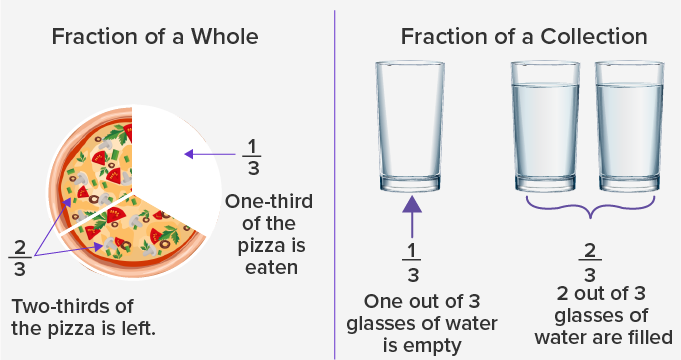A fraction consists of a Numerator and a Denominator.

The numerator tells how many parts out of all are there. The denominator tells how many total parts are there.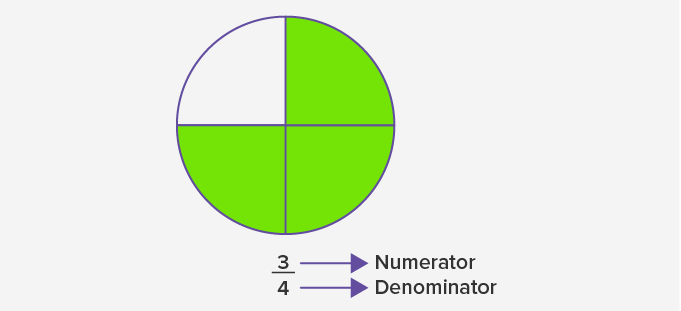## How to Use Improper Fraction:

A fraction in which the numerator is equal to or greater than the denominator is called an improper fraction.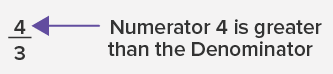But, how do we get an improper fraction?

Imagine you order a pizza which has 6 slices.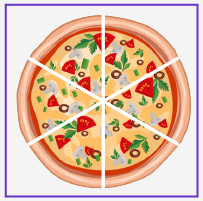Your friends eat all the 6 slices.And you realize you didn’t get any.You order another pizza.

After eating 1 slice from it you realize you are done eating.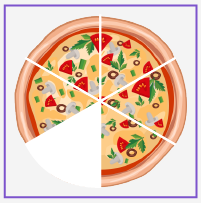So, how much of the pizza did your friends, and you have in all?

Your friends first had all the six slices of 1 pizza, and then you had 1 slice out of the size from the second pizza.

So, the total pizza eaten is 7slices of pizza. And that’s an improper fraction with a numerator greater than the denominator.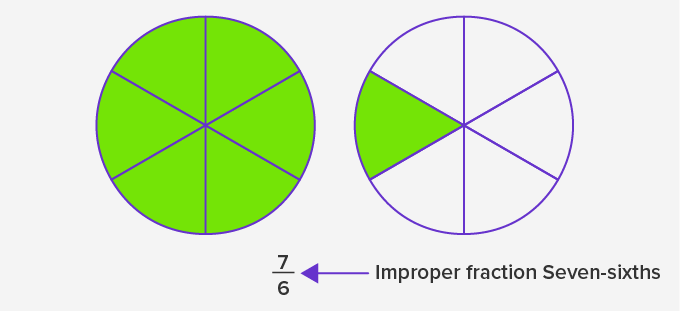## Types of Fractions:

There are 3 types of fractions are: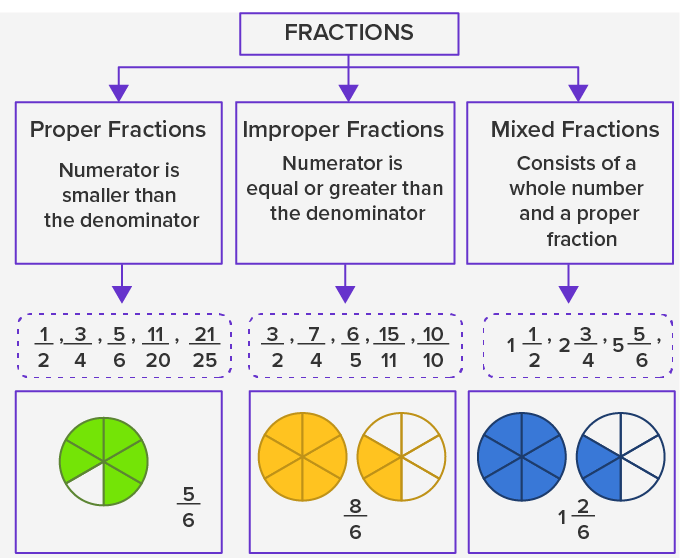Converting improper fraction to mixed fraction:

Follow the given steps to convert an improper fraction to a mixed fraction:

Example: Convert 7to a mixed fraction.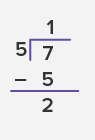Step 1: Divide the numerator by the denominator.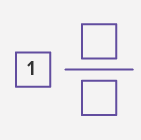Step 2: Write the quotient as the whole number part.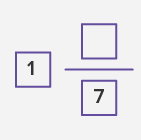Step 3: For the fractional part, keep the denominator the same.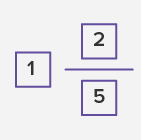Step 4: Write the remainder as the numerator.

Converting mixed fraction to improper fraction

Follow the given steps to convert an improper fraction to a mixed fraction:

Example: Convert 1 3to a mixed fraction.

Step 1: Multiply the denominator and the whole number.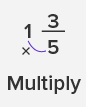Step 2: Add the numerator to the product of step 1.

We get: 5 × 1 + 3 = 5 + 3 = 8.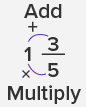This is the numerator.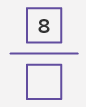Step 3: Keep the denominator the same.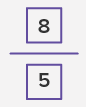Fun Fact: Improper fractions are easier to do calculations such as addition and subtraction, but mixed fractions are easier to understand.

Won Numerous Awards & Honors